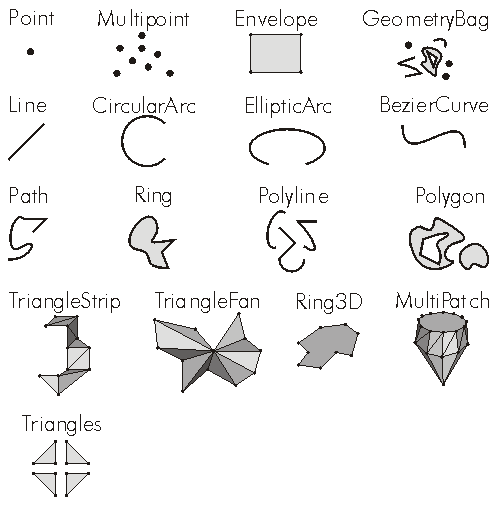ArcObjects Library Reference (Geometry)

# esriGeometryType Constants

The available kinds of geometry objects.

Constant Value Description
esriGeometryNull 0 A geometry of unknown type.
esriGeometryPoint 1 A single zero dimensional geometry.
esriGeometryMultipoint 2 An ordered collection of points.
esriGeometryLine 13 A straight line segment between two points.
esriGeometryCircularArc 14 A portion of the boundary of a circle.
esriGeometryEllipticArc 16 A portion of the boundary of an ellipse.
esriGeometryBezier3Curve 15 A third degree bezier curve (four control points).
esriGeometryPath 6 A connected sequence of segments.
esriGeometryPolyline 3 An ordered collection of paths.
esriGeometryRing 11 An area bounded by one closed path.
esriGeometryPolygon 4 A collection of rings ordered by their containment relationship.
esriGeometryEnvelope 5 A rectangle indicating the spatial extent of another geometry.
esriGeometryAny 7 Any of the geometry coclass types.
esriGeometryBag 17 A collection of geometries of arbitrary type.
esriGeometryMultiPatch 9 A collection of surface patches.
esriGeometryTriangleStrip 18 A surface patch of triangles defined by three consecutive points.
esriGeometryTriangleFan 19 A surface patch of triangles defined by the first point and two consecutive points.
esriGeometryRay 20 An infinite, one-directional line extending from an origin point.
esriGeometrySphere 21 A complete 3 dimensional sphere.
esriGeometryTriangles 22 A surface patch of triangles defined by non-overlapping sets of three consecutive points each.

#### Product Availability

Available with ArcGIS Engine, ArcGIS Desktop, and ArcGIS Server.

#### Remarks

A list of the distinct types of geometries creatable geometries.  Every geometry object belongs to and is identified as exactly one of these types (With the exception of esriGeometryAny which is true for all valid geometries.).

```esriGeometryNull          = Unknown type of geometry
esriGeometryPoint         = Point
esriGeometryMultipoint    = Multipoint (Collection of Points)
esriGeometryLine          = Line         (Segment)
esriGeometryCircularArc   = CircularArc  (Segment)
esriGeometryEllipticArc   = EllipticArc  (Segment)
esriGeometryBezier3Curve  = BezierCurve  (Segment)
esriGeometryPath          = Path
esriGeometryPolyline      = Polyline (Collection of Paths)
esriGeometryRing          = Ring (Ring / SurfacePatch)
esriGeometryPolygon       = Polygon  (Collection of Rings)
esriGeometryEnvelope      = Envelope
esriGeometryAny           = Any valid geometry
esriGeometryBag           = GeometryBag (Collection of Geometries)
esriGeometryMultiPatch    = MultiPatch  (Collection of SurfacePatches)
esriGeometryTriangleStrip = TriangleStrip (SurfacePatch)
esriGeometryTriangeFan    = TriangleFan   (SurfacePatch)
esriGeometryRay           = Ray
esriGeometrySphere        = Sphere
esriGeometryTriangles        = Triangles (SurfacePatch)
```
``````
[C#]

public static void GeometryToString(IGeometry geometry)

{

if (geometry == null)

{

Trace.WriteLine("Geometry Is Null.");

}

else

{

Trace.WriteLine("geometry.GeometryType = " + geometry.GeometryType);

if (geometry.IsEmpty)

{

Trace.WriteLine("Geometry Is Empty.");

}

else

{

switch (geometry.GeometryType)

{

case esriGeometryType.esriGeometryPoint:

IPoint point = geometry as IPoint;

Trace.WriteLine("point = " + PointToString(point));

break;

case esriGeometryType.esriGeometryRay:

IRay ray = geometry as IRay;

Trace.WriteLine("ray.Origin = " + PointToString(ray.Origin));

Trace.WriteLine("ray.Vector.XComponent = " + ray.Vector.XComponent);

Trace.WriteLine("ray.Vector.YComponent = " + ray.Vector.YComponent);

Trace.WriteLine("ray.Vector.ZComponent = " + ray.Vector.ZComponent);

Trace.WriteLine("ray.Vector.Magnitude = " + ray.Vector.Magnitude);

break;

case esriGeometryType.esriGeometryLine:

ILine line = geometry as ILine;

Trace.WriteLine("line.FromPoint = " + PointToString(line.FromPoint));

Trace.WriteLine("line.ToPoint = " + PointToString(line.ToPoint));

break;

case esriGeometryType.esriGeometryEnvelope:

IEnvelope envelope = geometry as IEnvelope;

Trace.WriteLine("envelope.XMin = " + envelope.XMin);

Trace.WriteLine("envelope.XMax = " + envelope.XMax);

Trace.WriteLine("envelope.YMin = " + envelope.YMin);

Trace.WriteLine("envelope.YMax = " + envelope.YMax);

Trace.WriteLine("envelope.ZMin = " + envelope.ZMin);

Trace.WriteLine("envelope.ZMax = " + envelope.ZMax);

break;

case esriGeometryType.esriGeometryPolyline:

IGeometryCollection geometryCollection = geometry as IGeometryCollection;

Trace.WriteLine("polyline.PathCount = " + geometryCollection.GeometryCount);

for (int i = 0; i < geometryCollection.GeometryCount; i++)

{

Trace.WriteLine("polyline.Path[" + i + "]");

IGeometry pathGeometry = geometryCollection.get_Geometry(i);

IPointCollection pathPointCollection = pathGeometry as IPointCollection;

for (int j = 0; j < pathPointCollection.PointCount; j++)

{

Trace.WriteLine("Point[" + j + "] = " + PointToString(pathPointCollection.get_Point(j)));

}

}

break;

case esriGeometryType.esriGeometryPolygon:

IPolygon4 polygon = geometry as IPolygon4;

IGeometryBag exteriorRingGeometryBag = polygon.ExteriorRingBag;

IGeometryCollection exteriorRingGeometryCollection = exteriorRingGeometryBag as IGeometryCollection;

Trace.WriteLine("polygon.ExteriorRingCount = " + exteriorRingGeometryCollection.GeometryCount);

for (int i = 0; i < exteriorRingGeometryCollection.GeometryCount; i++)

{

Trace.WriteLine("polygon.ExteriorRing[" + i + "]");

IGeometry exteriorRingGeometry = exteriorRingGeometryCollection.get_Geometry(i);

IPointCollection exteriorRingPointCollection = exteriorRingGeometry as IPointCollection;

for (int j = 0; j < exteriorRingPointCollection.PointCount; j++)

{

Trace.WriteLine("Point[" + j + "] = " + PointToString(exteriorRingPointCollection.get_Point(j)));

}

IGeometryBag interiorRingGeometryBag = polygon.get_InteriorRingBag(exteriorRingGeometry as IRing);

IGeometryCollection interiorRingGeometryCollection = interiorRingGeometryBag as IGeometryCollection;

Trace.WriteLine("polygon.InteriorRingCount[exteriorRing" + i + "] = " + interiorRingGeometryCollection.GeometryCount);

for (int k = 0; k < interiorRingGeometryCollection.GeometryCount; k++)

{

Trace.WriteLine("polygon.InteriorRing[" + k + "]");

IGeometry interiorRingGeometry = interiorRingGeometryCollection.get_Geometry(k);

IPointCollection interiorRingPointCollection = interiorRingGeometry as IPointCollection;

for (int m = 0; m < interiorRingPointCollection.PointCount; m++)

{

Trace.WriteLine("Point[" + m + "] = " + PointToString(interiorRingPointCollection.get_Point(m)));

}

}

}

break;

case esriGeometryType.esriGeometryMultiPatch:

IGeometryCollection multiPatchGeometryCollection = geometry as IGeometryCollection;

Trace.WriteLine("multiPatch.PartCount = " + multiPatchGeometryCollection.GeometryCount);

for (int i = 0; i < multiPatchGeometryCollection.GeometryCount; i++)

{

IGeometry partGeometry = multiPatchGeometryCollection.get_Geometry(i);

Trace.WriteLine("multiPatch.Part[" + i + "].geometryType = " + partGeometry.GeometryType);

IPointCollection partPointCollection = partGeometry as IPointCollection;

for (int j = 0; j < partPointCollection.PointCount; j++)

{

Trace.WriteLine("Point[" + j + "] = " + PointToString(partPointCollection.get_Point(j)));

}

}

break;

default:

IPointCollection pointCollection = geometry as IPointCollection;

for (int i = 0; i < pointCollection.PointCount; i++)

{

Trace.WriteLine("Point[" + i + "] = " + PointToString(pointCollection.get_Point(i)));

}

break;

}

}

}

Trace.WriteLine(null);

}

private static string PointToString(IPoint point)

{

return (point.X + ", " + point.Y + ", " + point.Z);

}